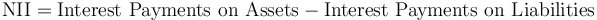# Net Interest Income

## Definition

Net Interest Income (NII) is an accounting term denoting the difference between Gross Income generated by interest-bearing banking assets (loans etc.) and the debt servicing cost of liabilities (loans, deposits, bonds).

NII is an absolute figure, using the unit of account (e.g. Euro). A relative measure is provided by the Net Interest Margin metric

## Formula$\mbox{NII} = \mbox{Interest Payments on Assets} - \mbox{Interest Payments on Liabilities}$